# VLOOKUP with IF statement in Excel

The tutorial shows how to combine VLOOKUP and IF function together to v-lookup with if condition in Excel. You will also learn how to use IF ISNA VLOOKUP formulas to replace #N/A errors with your own text, zero or blank cell.

Whilst the VLOOKUP and IF functions are useful on their own, together they deliver even more valuable experiences. This tutorial implies that you remember the syntax of the two functions well, otherwise you may want to brush up on your knowledge by following the above links.

## Vlookup with If statement: return True/False, Yes/No, etc.

One of the most common scenarios when you combine If and Vlookup together is to compare the value returned by Vlookup with a sample value and return Yes / No or True / False as the result.

In most cases, the following generic formula would work nicely:

IF(VLOOKUP(…) = sample_value, TRUE, FALSE)

Translated in plain English, the formula instructs Excel to return True if Vlookup is true (i.e. equal to the sample value). If Vlookup is false (not equal to the sample value), the formula returns False.

Below you will a find a few real-life uses of this IF Vlookup formula.

#### Example 1. Compare Vlookup result with a specific value

Let's say, you have a list of items in column A and quantity in column B. You are creating a dashboard for your users and need a formula that would check the quantity for an item in E1 and inform the user whether the item is in stock or sold out.

You pull the quantity with a regular Vlookup with exact match formula like this:

`=VLOOKUP(E1,\$A\$2:\$B\$10,2,FALSE)`

Then, write an IF statement that compares Vlookup's result with zero, and returns "No" if it is equal to 0, "Yes" otherwise:

`=IF(VLOOKUP(E1,\$A\$2:\$B\$10,2,FALSE)=0,"No","Yes")`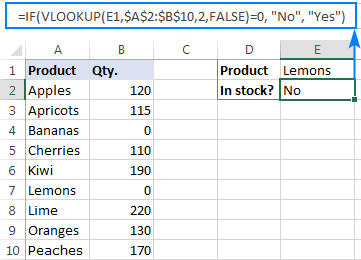Instead of Yes/No, you can return TRUE/FALSE or In Stock/Sold out or any other two choices. For example:

`=IF(VLOOKUP(E1,\$A\$2:\$B\$10,2)=0,"Sold out","In stock")`

You can also compare the value returned by Vlookup with sample text. In this case, be sure to enclose a text string in quotation marks, like this:

`=IF(VLOOKUP(E1,\$A\$2:\$B\$10,2)="sample text",TRUE,FALSE)`

#### Example 2. Compare Vlookup result with another cell

Another typical example of Vlookup with If condition in Excel is comparing the Vlookup output with a value in another cell.  For example, we can check if it's greater than or equal to a number in cell G2:

`=IF(VLOOKUP(E1,\$A\$2:\$B\$10,2)>=G2,"Yes!","No")`

And here is our If formula with Vlookup in action: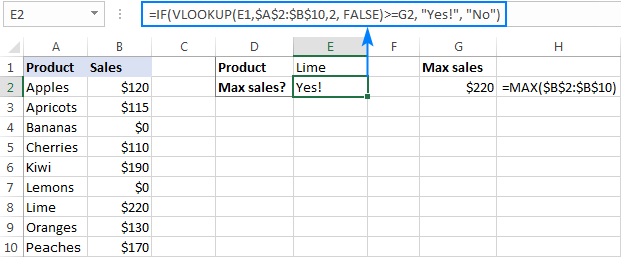In a similar fashion, you can use any other logical operator together with a cell reference in your Excel If Vlookup formula.

#### Example 3. Vlookup values in a shorter list

To compare each cell in the target column with another list and return True or Yes if a match is found, False or No otherwise, use this generic IF ISNA VLOOKUP formula:

IF( ISNA( VLOOKUP(…)),"No","Yes")

If Vlookup results in the #N/A error, the formula returns "No", meaning the lookup value is not found in the lookup list. If the match is found, "Yes" is returned. For example:

`=IF(ISNA(VLOOKUP(A2,\$D\$2:\$D\$4,1,FALSE)),"No","Yes")`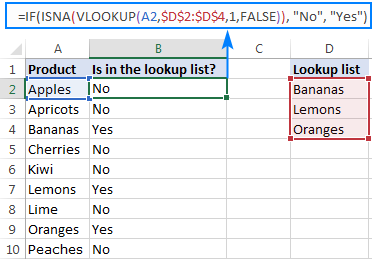If your business logic requires the opposite results, simply swap "Yes" and "No" to reverse the formula's logic:

`=IF(ISNA(VLOOKUP(A2,\$D\$2:\$D\$4,1,FALSE)),"Yes","No")`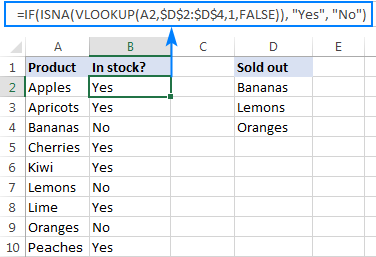## Excel If Vlookup formula to perform different calculations

Besides displaying your own text messages, If function with Vlookup can perform different calculations based on the criteria you specify.

Taking our example further, let's calculate the commission of a specific seller (F1) depending on their effectiveness: 20% commission for those who made \$200 and more, 10% for everyone else.

For this, you check if the value returned by Vlookup is greater than or equal to 200, and if it is, multiply it by 20%, otherwise by 10%:

`=IF(VLOOKUP(F1,\$A\$2:\$C\$10,3,FALSE )>=200, VLOOKUP(F1,\$A\$2:\$C\$10,3,FALSE)*20%, VLOOKUP(F1,\$A\$2:\$C\$10,3,FALSE)*10%)`

Where A2:A10 are seller names and C2:C10 are sales.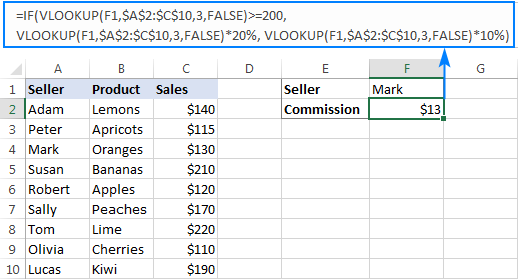## IF ISNA VLOOKUP to hide #N/A errors

If the VLOOKUP function cannot find a specified value, it throws an #N/A error.  To catch that error and replace it with your own text, embed a Vlookup formula in the logical test of the IF function, like this:

Supposing, you have a list of seller names in one column and sales amounts in another column. Your task is to pull a number corresponding to the name the user enters in F1. If the name is not found, display a message indicating so.

With the names in A2:A10 and amounts C2:C10, the task can be fulfilled with the following If Vlookup formula:

`=IF(ISNA(VLOOKUP(F1,\$A\$2:\$C\$10,3,FALSE)), "Not found", VLOOKUP(F1,\$A\$2:\$C\$10,3,FALSE))`

If the name is found, a corresponding sales amount is returned: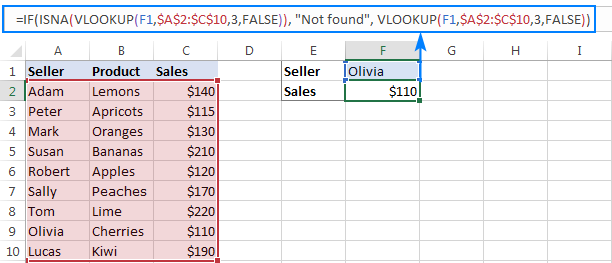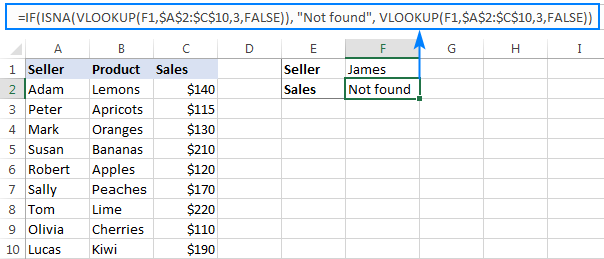#### How this formula works

The formula's logic is very simple: you use the ISNA function to check Vlookup for #N/A errors. If an error occurs, ISNA returns TRUE, otherwise FALSE. The above values go to the logical test of the IF function, which does one of the following:

• If the logical test is TRUE (#N/A error), your message is displayed.
• If the logical test is FALSE (lookup value is found), Vlookup returns a match normally.

## IFNA VLOOKUP in newer Excel versions

In Excel for Office 365, Excel 2019, Excel 2016 and 2013, you can use the IFNA function instead of IF ISNA to catch and handle #N/A errors:

In our example, the formula would take the following shape:

`=IFNA(VLOOKUP(F1,\$A\$2:\$C\$10,3, FALSE), "Not found")`

Tip. If you'd like to trap all sorts of errors, not only #N/A, use VLOOKUP in combination with the IFERROR function. More details can be found here: IFERROR VLOOKUP in Excel.

When working with numerical values, you may want to return a zero when the lookup value is not found. To have it done, use the IF ISNA VLOOKUP formula discussed above with a little modification: instead of a text message, supply 0 in the value_if_true argument of the IF function:

IF(ISNA(VLOOKUP(…)), 0, VLOOKUP(…))

In our sample table, the formula would go as follows:

`=IF(ISNA(VLOOKUP(F2,\$A\$2:\$C\$10,3,FALSE)), 0, VLOOKUP(F2,\$A\$2:\$C\$10,3,FALSE))`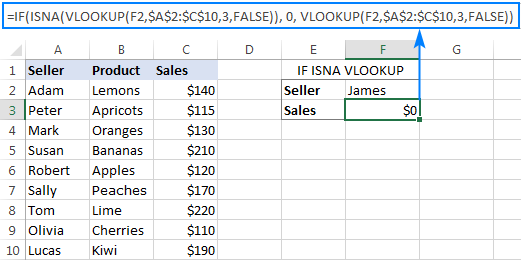In the recent versions of Excel 2016 and 2013, you can use the IFNA Vlookup combination again:

`=IFNA(VLOOKUP(I2,\$A\$2:\$C\$10,3, FALSE), 0)`

This is one more variation of the "Vlookup if then" statement: return nothing when the lookup value is not found. To do this, instruct your formula to return an empty string ("") instead of the #N/A error:

IF(ISNA(VLOOKUP(…)), "", VLOOKUP(…))

Below are a couple of complete formula examples:

For all Excel versions:

`=IF(ISNA(VLOOKUP(F2,\$A\$2:\$C\$10,3,FALSE)), "", VLOOKUP(F2,\$A\$2:\$C\$10,3,FALSE))`

For Excel 2016 and Excel 2013:

`=IFNA(VLOOKUP(F2,\$A\$2:\$C\$10,3, FALSE), "")`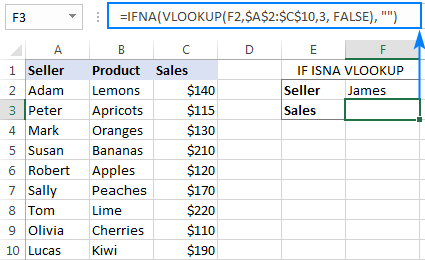## If with Index Match - left vlookup with If condition

Experienced Excel users know that the VLOOKUP function is not the only way to do vertical lookup in Excel. The INDEX MATCH combination can also be used for this purpose and it's even more powerful and versatile.  The good news is that Index Match can work together with IF in exactly the same way as Vlookup.

For example, you have order numbers in column A and seller names in column B. You are looking for a formula to pull the order number for a specific seller.

Vlookup cannot be used in this case because it cannot search from right to left. Index Match will work without a hitch as long as the lookup value is found in the lookup column. If not, a #N/A error will show up. To replace the standard error notation with your own text, nest Index Match inside IF ISNA:

`=IF(ISNA(INDEX(A2:A10, MATCH(F1, \$B\$2:\$B\$10, 0))), "Not found", INDEX(A2:A10, MATCH(F1, \$B\$2:\$B\$10, 0)))`

In Excel 2016 and 2016, you can use IFNA instead of IF ISNA to make the formula more compact:

`=IFNA(INDEX(A2:A10, MATCH(F1, \$B\$2:\$B\$10, 0)), "Not found")`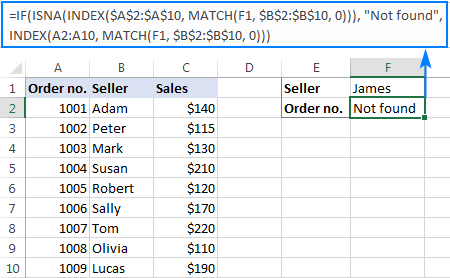In a similar manner, you can use Index Match in other If formulas.

This is how you use Vlookup and If statement together in Excel. To have a closer look at the formulas discussed in this tutorial, you are welcome to download our sample workbook to Excel If Vlookup. I thank you for reading and hope to see you on our blog next week!

### 86 Responses to "VLOOKUP with IF statement in Excel"

1.Harish says:

Doubt in google Excel sheet Formula:

I created two sheets. sheet one contains full data base with date and name , In sheet2 I have form submission lead , That is date and name with other data's. Here i need to make display other data in sheet 2 related to the particular Date and name from sheet 1.

•Gennady Terekhov (Ablebits.com Team) says:

Hello,

For us to understand your task better, we need to look at your data. If it is possible, please share your workbook with the source data and the expected result with support@ablebits.com. Please shorten the tables to 10-20 rows/columns and do not forget to include the link to your blog comment in the email.

2.Nicholas says:

Hi Svetlana,
I have a problem.
I have two spreadsheets. Spreadsheet "A" has a list of part numbers and sell prices. Spreadsheet "B" has the same part numbers plus more which spreadsheet "A"does not have - including prices.
When I populate prices from SSHeet "A" to SSheet "B" using VLookup, where there is no data from Ssheet "A", I naturally return a #N/A. So what I want to do is somehow create a Vlookup formula where I can also include a formula which will use another cell in SSheet "B" where I have a cost of product and apply a margin to that cost and do this all within the VLOOKUP formula. Is this possible?
Thanks Svetlana.

•Svetlana Cheusheva (Ablebits.com Team) says:

Hi Nicholas,

Thank you for your kind words!

I do not exactly understand the part where you apply a margin to the cost, but I believe the general idea is feasible. You coulod nest IFERROR within VLOOKUP as shown in this example.

•Kjell Eliasson says:

Hi , I saw your solutions and im impressed, maybe you can help me as well ..

I have question :-)

I use =IF(I3="SE",VLOOKUP(B24,ls_all_courses,7,0)) to find a numeric value (Column 7)

But i want to add more choices with IF , IF(I3="XX",VLOOKUP(B24,ls_all_courses,8,0

So if it is XX instead of SE , the column should be 8

Is this possible or do i need to use another formula ?

Kjell

•Muhammad Nadeem says:

HI SVETLANA,
i have a problem during applying of v lookup i have two sheets which are the first sheet only material description and second one have material code and description i want to apply material code in first sheet but during this only applied single description while came duplicate description the formula shown #NA.please suggest.
Image:
=VLOOKUP(G3,Sheet1!D1:E965,2,FALSE)
HIGHLIGHTER 6000000026
HIGHLIGHTER #N/A

3.Shrikant says:

Hello Svetlana,

I have calculated a 'x' value in a cell by using formula.
Now I have to use that 'x' value in vlookup by giving cell address but it is showing error #NA.
Could you please help me out. I want vlookup to use the value from a particular cell address which is calculated by a formula to show desired result.

-Regards,
Shrikant

•Svetlana Cheusheva (Ablebits.com Team) says:

Hi Shrikant,

Normally, you simply supply a cell reference instead of the lookup value in the first argument, e.g. =VLOOKUP(D1,A1:C10,3,FALSE)

It's hard to say why you are getting the #N/A error without seeing your data. Can you post both of your formulas here, the one that calculates "x" and Vlookup?

4.Stuart says:

Hi,

Thanks for the help, is it possible to, when the vlookup finds a match it then displays the data in another column on the same row as the matched data? essentially I have a two lists of orders and have compared the two to find orders that have doubled up.
What I used was
=IF(ISNA(VLOOKUP([@ID],'Sheet2'!E:E,5,FALSE)),"","Found")
At them moment I have to then find the entry and manually find the dates to see when the double up occurred, if i could automate that it would save me a bunch of time.
-Thanks,
Stuart

5.Abdur Rehman says:

=IFERROR(VLOOKUP(\$G\$14&\$K\$14&\$O\$14&\$R\$14&\$D19&\$E19,'RAW DATA'!\$A\$1:\$AB\$8000,F\$16,FALSE),"")

=IF(\$K\$14="NUMERIC DISTRIBUTION %",TEXT(F19/100,"0.00%"),F19)

i want to merge both formula in cell F19. can it possible...??
In second formula if i select another option replacement of "NUMERIC DISTRIBUTION %" (K14 is validation list) than run only vlookup formula.

6.natarajtn says:

if with vlookup for Value based or % not Text So. 1 to 1000 = 5%, 1001 to 2000 = 15%, 2001 to 5000 = 18%. 5001 and above 20% Interest or Tax like calculations required

7.Adewale says:

How is it possible to use vlookup for drop down and also insert if formula in the drop down

8.rattana warn says:

Why my VLOOKUP does not show the result, though I can see the formular,when I click in the cell

9.Mikee says:

Hello everyone,

Can someone help me with a formula (not sure if its even possible). I'm trying to create a tracker of lets say i choose an option from a drop down menu in E9, i want all the Cells From F9 to N9 to automatically populate(I figured how to do this already), but what i'm really trying to figure out is if there is a way to have cell F9 (if blank) to insert a date 1 year before a date in cell C9. Hope i'm making sense.

Thank you.

10.Mike says:

Hi,
We are a small manufacturing company in Texas. I verify material receiving dates against PO due dates with the falling formula. A spreadsheet for receiving and one for purchase orders. The problem is if the due date field in the PO spreadsheet doesn't have a date, then it comes back with a "N". Making it appear that we did not receive it in time. I was wanting to only target the dates in the PO master (due dates) that have a date in the cell. I've been trying to get everyone to put in an appropriate due date, but not everyone wants to get on board. Any help would be greatly appreciated.
Thank you,
Mike

=IF(A3977<=VLOOKUP(C3977,'[Purchase Order Master.xls]Sheet1'!\$A:\$J,8,0),"Y","N")

11.Thomas says:

Is there a way to choose which data is displayed with the IF formula based on the text in a certain column? Example: If column A is red it will display the information from column B, but if column A is any other color it will display information from column C?

12.molu says:

I want a multiple argument in my vlookup as follows:
Do it on the Required Field (as the only field) and the logic is:
1. If the vlookup finds a match, then return the value in the range
ELSE
2. If it does not find a match but the values of 2 separate fields are X1 = ABC and xx1 = DEF & XYZ, then return R
3. In all other cases where it does not find a match, then return NR

I already have the vlookup to return the value else NR - see below:

=IFNA(VLOOKUP(B9,H\$1:I\$2112,2,FALSE),"NR")

13.VELA says:

A IF the value is Equel to or above then 85 the payout shoud be 500
b IF the value is Equel to or above then 90 the payout shoud be 800
C IF the value is Below then 850 the payout shoud be 0

Please show me how to fix it by using IF.??

•Aman says:

Try this expression:

=IF(Value>=85,"500",IF(Value>=90,"800",IF(Value<850,"0")))

14.Wel says:

Hello -

Need help. Need to do vlookup for spreadsheet containing countries and accounts codes. How can i match the account code in each countries. I need to get the correct mapping of Final account for France and same with other countries.

Sample:Raw Data
Country Account Code
France 50152
Spain 50152
Italy 50152

Master File:

Country Account Code Final Account
France 50152 50155
Spain 50152 50140
Italy 50152 50150

15.Chris says:

Hello,

I've created a spreadsheet across 3 tabs which shows performance data from a team of almost 40 people.

What I have been asked to do is create a separate tab whereby if we enter a name within a certain cell, all of their individual data appears from the various tabs. Can someone point me in the right direction to making this a reality? I'm a novice when it comes to Excel but can pick things up quickly enough if given a sense of direction...

•Doug says:

Chris:
I would try the VLOOKUP and see how that works. You can use the INDEX MATCH option as well.
The article, "Excel VLOOKUP tutorial for beginners with formula examples" here on AbleBits would be a good place for you to start. In your case the VLOOKUP from another worksheet might be the way to go.

16.Deepak says:

Hi,

Suppose if the value of column A = " exact as X"and value of column B="exact as Y", formula should result the value of Column C.

•Doug says:

Deepak:
Where the sum of column A is in A10 and the sum of column B is in B10 and the value you want to display is in C10 the formula in an empty cell is:
=IF(AND(A10="exact as X",B10="exact as Y")C10,"No Value"))

17.Darren says:

There are great examples on here which I have used but struggling with something I am not sure is possible.
I need a vlookup with an if that continues down the list.

So if this was the data
A
1Tyre On site
Tyre Warehouse
Engine Germany

18.Darren says:

Sorry pressed enter by mistake.In simple terms I need a formula that looks up an entry and if there is also specific text in the same row returns a YES.

It only seems to work on the first hit in the list.

I have this formula in

F2

=IF(VLOOKUP(E1,\$A\$2:\$B\$9,2,FALSE)="Warehouse","YES","NO")

and this in f3

=IF(VLOOKUP(E1,\$A\$2:\$B\$9,2,FALSE)="onsite","YES","NO")

The word tyre in e1 !

But issue

M y first line has A2 B2 has
Tyre Warehouse the formula returns YES Which is correct

If in my second A3 B3 it has

Tyre onsite

This returns a NO which is incorrect.

If I go to the cell above A2 where it says "Tyre" and BT is "warehouse" and delete TYRE it then returns YES against "onsite" formula .

It like it stops searching after the first hit

on tyre warehouse

In reality both those formulas should present a YES

I basically want to look up a value in a table and if a entry on the same row is a specific piece of text give me a yes.

The value may be repeated several times the text will be one of a few things.

I appreciate I will need a formula per entry "warehouse", "onsite .

Any help would be appreciated.

•Doug says:

Darren:
I think you'll be better off using INDEX.
I built a little sample sheet using your data and I had some fun with it.
So, where the location data is in D22:D33, the items are in C22:C33 and the item you're looking for is in H22, the formula looks like this:
=INDEX(\$D\$22:\$D\$33,SMALL(IF(\$H22=\$C\$22:\$C\$33,ROW(\$C\$22:\$C\$33)-ROW(\$C\$22)+1),1))
There are a few things to note about this. First off it is an array formula so when you enter it into the formula bar put the cursor in the formula somewhere and click CTRL+Shift+Enter at the same time. When you do you'll see the curly brackets around the entire formula.
Second, the last "1" in the formula is telling Excel which instance of the item in the list you want to return. If you change the "1" to "2" the items second instance in the list will be displayed. Change the "2" to "3" and the items third instance will be displayed.
This will be useful if you enter "First Location" in cell I22 and "Second Location" in I23 and so on. Then enter the formula in H22 with the "1" and enter the formula with a "2" in H23 and so on. You're returning the location of each of the items. This way each of the items location can be displayed by selecting the items instance in the list. You can show where the Tyre is in every location. 1 might be warehouse, 2 might be Germany, 3 might be On-site, etc.

19.Darren says:

Finally got back round to looking at it.

Thanks Doug I would of never thought of that formula at all !. I have done what you said and edited the +1 to +2 etc, and it continues down the list so thanks ever so much for the help.

Daz

20.Brian Sutowski says:

I'll begin by saying I am relatively new to using Excel (~1 YR).

I basically need to do the following:

I have a list of about 10K different items that we sell that I use the data sort function to sort by A) total units sold, B) # of months the item sold in the past year, and C) total number of hits (sales order lines)

Essentially the exact same items in three different lists (or one list and two tables) in different order.

Basically I want to take the top 2000 items by units sold and use vlookup to find matches in the top 2000 items in the other two list sorts.

I am thinking along the lines of a IF vlookup table1 AND vlookup table2 -- but I am not familiar enough to input the appropriate formula.

Thanks!

~Brian

21.Jennifer says:

I'm not sure if this is possible, but figured I would ask. I have 1 column (354 rows) that include a unique query string in each and want to search that string for an account number (5-6 digits long). I have another column that lists all the possible account numbers to do a lookup against. Next to that column, I have a column with account names for each account number. I'm looking to see if it's possible in new column to return the account name associated with particular account number IF that account number is found in the query string. Let me know if I explained what I'm trying to do correctly and if this is possible without parsing out the account number from the long query string.

22.Nabilah Hannani says:

Hello, I have problem to merge my data, can you help me, please? Can I get your email to send you my data? Thank you :)

23.Andrew says:

Good afternoon,
I have emailed support@ablebits.com with a file (AJB Test Data) that I need help with please. I currently have a long IF calculation running over many columns to obtain the correct data that I need, but there must be a shorter way of doing it using Vlookup or Index Match maybe? In the Data Tab I need to use columns A and B to look up the corresponding column and cell on the Data 2 tab, then place the answer in the Data Tab, column C against the corresponding row. In essence there are two cells to match up to locate the correct data. In my working copy there may be up to three cells to match! Help would be greatly appreciated. Thank you.

24.Damon says:

In Excel, I have 2 sheets. One is titled "Equipment" and the other is "Technicians". What I would like to do is, on the Technicians sheet, In cell F3, I would like it to look on sheet "Equipment" from the range of I2:I17 and look for Gene? If Gene is found, then display the data from the corresponding cell in range A2:A17. This should be displayed in cell F3 on Technicians sheet. Any help would be appreciated!

25.Leonard Katongole says:

How can I use the if and vlookup formula using multiple data tables to return a "Yes" or "No" answer

26.Leonard Katongole says:

I have a main dataset of about 500,000 line items. I want to lookup the items from 3 different datasets. Each of these datasets has got about 200,000 line items. I want to use the if and vlookup function to return a " Yes" or "No" answer.

27.Arvind Sharma says:

I am looking for a value as below

look up the cell value =3300,0,VLOOKUP(N74,'Pay As You Earn'!\$A:\$N,14))

above formula is giving the correct value up to 12785.00
but after that its giving value 0

2) =IF(VLOOKUP(N75,'Pay As You Earn'!\$A:\$N,14,0)<=3300.01,0,VLOOKUP(N75,'Pay As You Earn'!\$A:\$N,14))

Where as the above formula is giving value 0 up to 12785.00
but after that its giving the correct value

please see and inform the reason if you want i can share the excel workbook for finding exact cause.

Thanks.

28.Arvind Sharma says:

sorry my question was like this

I am looking for a value as below

look up the cell value =3300.01,0,VLOOKUP(N74,'Pay As You Earn'!\$A:\$N,14))

above formula is giving the correct value up to 12,785.00
but amount beyond 12,785.00 that its giving value 0

and

2) =IF(VLOOKUP(N75,'Pay As You Earn'!\$A:\$N,14,0)<=3300.01,0,VLOOKUP(N75,'Pay As You Earn'!\$A:\$N,14))

Where as the above formula is giving correct value beyond amount 12785.00
but below amount 12785.00 its giving 0

please see and inform the reason if you want i can share the excel workbook for finding exact cause.

Thanks.

29.wz says:

Hi

How do i use the VLOOKUP and IF function to help me calculate my table whereby if a duplicate value is present then the function will help to average out these duplicate values based on the number of duplicate values there is e.g ( 3 & 3 = 3/2=1.5 , 3,3,3 = 3/3 = 1)

30.teena says:

Hi i am trying to set up a vlookup conditional formula where if value is >=1 it should add 5 to the value and if it is 0 , then it should return 0 but i tried to set a =if(vlookup.....)formula and for 0 it is returning #N/A, Please help

31.David P says:

Hello,

I have a question for you. Let's say I have the following content:

Cell A1: "I love puppies"
Cell A2: "I am a fan of dogs"
Cell A3: "Cats are awesome"
Cell A4: "Kittens are my favorite"

I want a formula that will see if a cell contains the words "puppies" and "dogs" and return the result "Dog Fan"
AND
I want the formula to see if a cell contains the words "Cats" and "Kittens" and return the result "Cat Fan" - how do I do this?

32.Rossa says:

Hi,

Could we do 'IF combined VLOOKUP' search a lot of data where located from more than one tabs? if possible how do I do this?

•Svetlana Cheusheva (Ablebits.com Team) says:

Hi Rossa,

It sounds like you need to do sequential, or chained, Vlookups. This can be done by nesting several IFERROR VLOOKUP formulas into each other. An example can be found here: How to do sequential Vlookups in Excel.

33.Muhammad Nehal says:

Hello!
i am having problems in using following formula, condition is True we get result correct but condition is False we get error. kindly help, pl.

IF(S2=VLOOKUP(S2,Reference!\$Q\$9:\$R\$28,1,0),VLOOKUP(S2,Reference!\$Q\$9:\$R\$28,2,0),N2)

34.mohammad says:

Thanks
good examples

35.Tasha says:

Hi. I have two spreadsheets with a list of more than 4k accounts each. I use vlookup with conditional formatting to determine if the same account number is on both sheets. But what I also need to do is, once it is established that the account number is on both sheets, update the balance on one sheet to the other on each account that is matched. Can you help me?

36.aly says:

I want to compare two columns for comparing students if there is new admission or left school. I need the result in following month in a separate column. That column should contain three words: Enrolled in previous month(if found in both months), new addmission( if found unique), SLC/Struck Off(if not found at all in following month). I need to know the new entrants and leavers, so that I add and subtract these with opening strength(previous month) to get closing strength balance. Duplicate conditional highlights and leaves unqiue,but those who left the school will not be at all in the following month(second column). but it is of no use for me. If any vlookup with three condition is there, it will be fine.

37.aly says:

thanks rajesh peshiya for your interest in my problem solution,below is format of my data and requirement.
SID-Sept SID-Oct (comparison)"

10910 10910 Existing Student
9116 9116 Existing Student
11229 11229 Existing Student
11769 11769 Existing Student
Stuck Off
Stuck Off
Stuck Off

Common means old student
unirque in Sept means new addmission

My requirement Summary
Opening Strenght(1-10-18) 7
Less S/Off -3
Closing Strength(30-10-18) 9

38.Pradeep says:

Hi,
I have query regarding find/search and vlookup.
I want find specific text from cell (string) and retrieve data from vlookup table by using this specific text.
e.g. “This is a boy” or “This is a girl” is in one cell.(there will more than 100 sentences) In Vlookup table Boy = Male and girl = Female.
I want display Male or Female in another cell of same sheet depends on cell string contains boy or girl.
Regards,

39.Isabel says:

I have a list of sales with sold date for different months.
I need a formula to pull sales by month.
any ideas?

40.tamil says:

Hi...
I have list of employees details with unique employee id in one sheet and in another sheet i have only employee id. If i type Resigned against one worker in sheet 1 means that workers code should be highlighted in sheets 2..pls suggest any formulas

41.Magnus Berge says:

Hello

I am trying to compare vlookup values in order to insert up or down arrows or equal sign based on these conditions. I have used the following formula but it doesnt work:

=IF(VLOOKUP(\$C\$2,'INTL FOB-CFR FOREX'!\$A\$1:\$SO\$965,7,TRUE)>VLOOKUP(\$C\$2-7,'INTL FOB-CFR FOREX'!\$A\$1:\$SO\$965,7,TRUE),"▲"),IF(VLOOKUP(\$C\$2,'INTL FOB-CFR FOREX'!\$A\$1:\$SO\$965,7,TRUE)<VLOOKUP(\$C\$2-7,'INTL FOB-CFR FOREX'!\$A\$1:\$SO\$965,7,TRUE),"▼"),IF(VLOOKUP(\$C\$2,'INTL FOB-CFR FOREX'!\$A\$1:\$SO\$965,7,TRUE)=VLOOKUP(\$C\$2-7,'INTL FOB-CFR FOREX'!\$A\$1:\$SO\$965,7,TRUE),"=")

Best regards,
Magnus

•Natalia Sharashova (Ablebits.com Team) says:

Hello, Magnus,

I'm sorry, it's rather difficult to correct your formula since it misses a few important parts:

1) The first argument of the Vlookup function cannot be a range (\$C\$2-7). It should be either a value or a single cell reference. Also, the correct data range should be written like \$C\$2:\$C\$7.
You can learn the basics of Vlookup here.
2) Also, you entered excess closing brackets after each "▼" that break you nested IF. Please refer to this article to check what arguments IF and nested IF contain.
3) The very last part of the formula misses the third argument after "=" that would indicate what to return if none condition is met.

Please consider these points when fixing the formula.

•Magnus Berge says:

Dear Natalie,

Many thanks! I have removed the brackets and the formula now works! The '\$C\$2' is a weekly date reference and the '\$C\$2-7' to get the previous week value.

The formula now reads as follow:

=IF(VLOOKUP(\$C\$2,'INTL FOB-CFR FOREX'!\$A\$1:\$SO\$965,31,TRUE)>VLOOKUP(\$C\$2-7,'INTL FOB-CFR FOREX'!\$A\$1:\$SO\$965,31,TRUE),"▲",IF(VLOOKUP(\$C\$2,'INTL FOB-CFR FOREX'!\$A\$1:\$SO\$965,31,TRUE)<VLOOKUP(\$C\$2-7,'INTL FOB-CFR FOREX'!\$A\$1:\$SO\$965,31,TRUE),"▼",IF(VLOOKUP(\$C\$2,'INTL FOB-CFR FOREX'!\$A\$1:\$SO\$965,31,TRUE)=VLOOKUP(\$C\$2-7,'INTL FOB-CFR FOREX'!\$A\$1:\$SO\$965,31,TRUE),"=")))

Thank you again.

Best regards,
Magnus Berge

•Natalia Sharashova (Ablebits.com Team) says:

You're most welcome, Magnus,

Now I've got those parts of your formula as well, thanks for the explanation!
Anyway, glad to know my suggestion worked! :)

•Magnus Berge says:

Thank you again, Natalia.

Happy New Year!

Best,
Magnus

•Natalia Sharashova (Ablebits.com Team) says:

Happy New Year, Magnus! :)

42.Binod Sharma says:

I have origin as mumbai and destination as a ahmedabad and
Origin Dst Destination Base Fuel Distance

43.Dhanushka says:

Hi I need a how to applyy comdition& formulass for one equation /one bracaket)

44.Vin says:

Hi All, Please kindly help me check if the below formula using if and vlookup is wrong?
=IF(VLOOKUP(A5,A2:A4,1,FALSE)=0,"No","Yes")
I got the outcome of the vlookup value as yes which is correct but for value that were not found, I`m getting as #N/A instead of the suppose return value of "No"

45.Workg says:

How to combine this 2 formula?
Need help.
=IF(LEFT(E2,1)="B","YES",IF(LEFT(E2,1)="W","YES","NO"))
and
=IF(L2="B999","Y",IF(L2="W999","Y",IF(L2="DB10","Y",IF(L2="RC10","Y",IF(L2="TTST","Y",IF(L2="UTST","Y",IF(L2="USTS","Y","N")))))))

46.jp says:

looking to create a spreadsheet with some complexity with user input and data supplied.
Willing to pay for its creation, but need to discuss with someone for details? what is the best forum to find someone?

47.Rasha says:

Hi
I have two sheets one is for staff another for day request
In the first sheet for "staff" column for 'Leave Type'such as sick leave or vacation leave, and another 'start date'the date of starting of the leave
In the second sheet, there is a cell "Date of presenting the leave"
How I can in the cell of Date of presenting the leave, write formula to calculate the following ;
if the type of the leave is vacation leave the date will be before 3 days from the date of this leave which existing in column of 'start date'for this leave
and if the type of the leave is Sick leave the date will be after 3 days from the date of this leave which existing in column of 'start date'for this leave

48.RAJ KUMAR says:

1000 KA 3% =30 (MAXIMUM 25 YA MINIMUM 3%)

49.mohit says:

i want use VLOOKUP between tow dates like i have January month data but from there need to pick only 15 January to 25 January data as per dates...

50.Ed Parry says:

Hi,
I am using a VLOOKUP function to find a value form a different tab but I need to replace what it finds with a different description i.e. When it finds "Steel Drum" I need that to be replaced with "Type 2 Drum".
Any help would be appreciated.
Many thanks
Ed

•Julie says:

Hi. Try

=IF(VLOOKUP(D20,R:S,2,FALSE)="steel drum",("type 2 drum"),(""))

Just replace
D20 with the value you're looking for
R:S the 2 columns where you're looking from
the end "" if you want it to say anything else

51.Qasim Mustafa Ali says:

I want an if statment Vlookup that instead of replacing the N/A it simply wouldn't change it at all.

For example I have pending, declined, approved.

I want a Vlookup to change from Pending to Approved or Declined but sometimes we update the rest on the second day. So I would want which wasn't found to be as it is and the Vlookup doesn't change it. Is that possible?

52.Peshiya Boy says:

Hello Team,
I have attendance data of 4 years. i want to see the summary of one person, so i am looking any formula which can take the desire value from that sheet.

53.Julie says:

I have 2 IF formulas that I need to perform on 1 cell to determine the outcome, how do I combine them into one so if the first one applies then do this but if it doesn't then do the second? These are the 2 formulas that work independently

(IF(VLOOKUP(B2,'AT Import'!C:H,6,false)="NP",(VLOOKUP(('Client allocation'!B2,'AT Import'!C:D,2,FALSE)-365),(VLOOKUP('Client allocation'!B2,'AT Import'!C:D,2,FALSE)))

IF(VLOOKUP(B2,'AT Import'!C:I,7,FALSE)="ok",(VLOOKUP('Client allocation'!B2,'AT Import'!C:D,2,FALSE)+365),(VLOOKUP('Client allocation'!B2,'AT Import'!C:D,2,FALSE)))

It's driving me nuts thanks!

54.TAHIR says:

Can somebody help me to find a formula for the items ("A,B,C") of column a .e.g,
if the same items in column a have dates in column b for all the respective cells, should give result complete otherwise incomplete.
Column (a) Column (b) Result Column (c)
A 12-Apr Completed
A 13-Apr Completed
B 13-Apr Incompleted
B Incompleted
C Incompleted
C Incompleted
C Incompleted
C Incompleted

55.Arif jan says:

A B C E F G
a MDF 15 10 a MDF 15
b MDF 10 5 b MDF9

kindly who used vlookup if Clom A and Clom E consider match and B and F apply vlookup

56.Marvin de Haas says:

I am trying to make this comparison check work in Excel 2016

I search across several tabs and I compare D6 with K6 and if they are the same then I want to show the value of D6 however I receive the #VALUE! error indicating that a value used in the formula is of a wrong data type, I tried to change values from text to numbers but have the same result any suggestions?

57.Priyanganie says:

Hi..
I have the formula as IF(P3="CSCL_REV","=vlookup(E3,'[Profit_&_Loss_Detail_Report AFKO.xlsx]AFKO'!\$B:\$E,4,0",0). What I want to get here is if P3="CSCL_REV", a value from a vlookup function. But this is not working & returning the same vlookup function if it is true. Thanks in advance.

58.Khalid says:

Use simple conditional formatting a simple condition make false disappear make condition if there is false then text color is white.

59.Sarah says:

I have a situation when I have students who have taken a test, and if they got 70 or above, I want it to say "HIS." However, there are many students who did not take the test at all or did not get 70 or above and in both of these instances, I want it to return blank.

Currently, I have =IF(VLOOKUP(A:A,'co 2024 (2)'!A:AC,29,false)>=70,"HIS",""). However, the blanks on the "Co 2024" tab are still coming up as HIS, even though I ideally would like them to come up as blanks. Can anyone help me out here?

60.Elango says:

I have data sheet with id status and I working same workbook another sheet 2. while enter the data id status is terminate want to highlight automaticaly

61.satish rane says:

I have three receipt date against one material code say X and in another excel file against material X i want to pick up latest date how can i do this
e.g.
material code - receipt date
X - 3-Sept-2019
X - 14-Aug-2019
X - 14-oct-2019

Now i want to pick up latest date i.e. 14-oct-2019 against material X

which formula to use

Data is huge and in above case, i just gave you one sample.

62.Teah Cruz says:

can this combination work for date formulas? i would like to create multiple formulas to find a list or sequence of dates to match any one person's payday. so that we dont have to rely on making a mistake on a paper calendar. so if someone gets paid weekly, bi weekly, semi monthly, on first of the month, or even on the 2nd, 3rd or 4th wednesday of the month.

63.Altaf Hussain says:

Sir/mam i need your help. I have a google sheet with of option chain data of multiple stocks. For each stock there are multiple strike prices. I need to get specific strike's premium when i put stock name in a cell. I tried to use IF and VLOOKUP together i got the results but the problem is,there are 70 stocks so i have to write the formula for each stocks that made it very very lenthy and time consuming. Please guide me. Thank you...

64.Kristen Becker says:

Can you tell me what is wrong with my formula? If my 'if statement' is true, I get the appropriate response of 62494, however, if my 'if statement' is false, my vlookup is giving me a #ref! error?

65.Kristen Becker says:

Can you tell me what is wrong with my formula? If my 'if statement' is true, I get the appropriate response of 62494, however, if my 'if statement' is false, my vlookup is giving me a #ref! error? UGH... helps if I include the function formula... sorry :) It's below:
=IF('Travel Expense Voucher'!\$F\$5=2,62494,VLOOKUP('Travel Expense Voucher'!M15,'Tcodes and Ecodes'!C11:D12,'Tcodes and Ecodes'!D11:D12,FALSE))

66.Raghupathi says:

How to add vlookup along with below formula
=IFERROR(IF(AND(N5>\$AN\$2,N5<\$AO\$2),N5,"-"),0)

67.Luke says:

I need to return a text based on the result found in the VLOOKUP function on the other tab.
=IF(A2="","-",VLOOKUP(A2,'Current RP - RQTY (IMS)'!A\$1:F\$5000,5,FALSE))
If the following are found this is what I need returned to the cell... Can anyone help me out of the ditch on this one?
1 = RawMaterial
2 = Formula
3 = Container
4 = Substrate

68.Arj says:

I'm trying to add an IF VLOOKUP with multiple search parameters - the idea I had was the following:

=IFERROR(VLOOKUP([@[LOCATION_NAME]],'SP Locations'!A:B,2,0)=No,VLOOKUP([@[LOCATION_NAME]],'SP Locations'!A:E,5,0)),
IFERROR(VLOOKUP([@[LOCATION_NAME]],'SP Locations'!A:B,2,0)=Yes,VLOOKUP([@[LOCATION_NAME]]&[@DEPTID],'SP Locations'!C:E,3,0))

I was wondering if it was at all possible to string the two IF searches together?

69.me says:

=IF('20182019EmpSW'!\$A\$2:\$A\$122246="2019Ogos",VLOOKUP(\$A\$5:\$A\$9000,'20182019EmpSW'!\$B\$2:\$AA\$122246,17,FALSE),VLOOKUP(\$A\$5:\$A\$9000,'20182019EmpSW'!\$B\$2:\$AA\$122246,18,FALSE))

70.Kim says:

I need to use a formula to look up a value in a column (we'll call this #1), based on the value in another column (#2), and then finally return the value in the column to the left of #1 based on whether it not it matches a certain word. Would vlookup or index match work better?

60+ professional tools for Excel